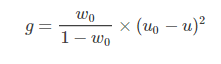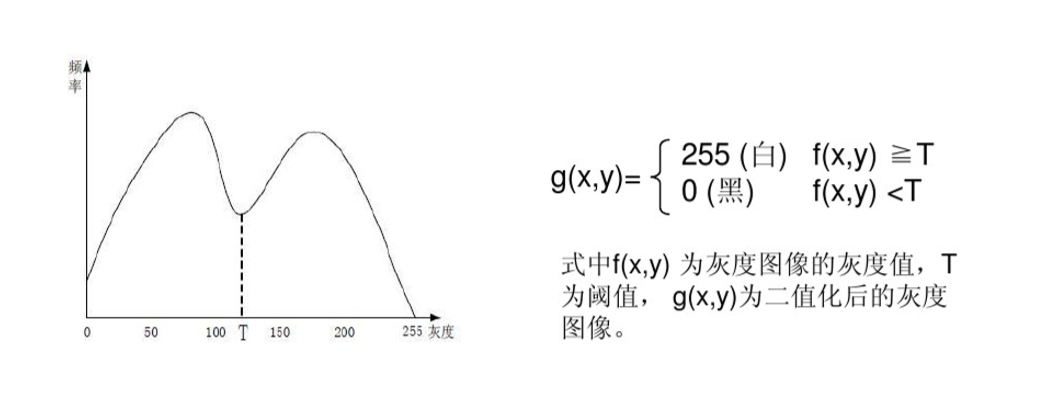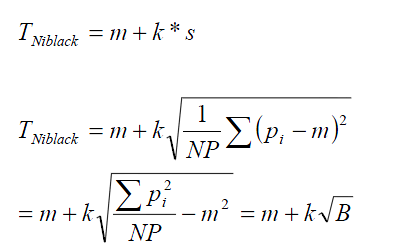图像处理中的二值化算法_什么叫二值化图像处理算法 - CSDN
• 上一节我们讲解了什么是二值化，并且讲到了二值化的一般方法，那么每种算法究竟是怎么样对图像经行二值化处理的呢？，算法的原理是什么呢，怎么样用代码实现，这节我们分享下。 1.otsu（最大类间方差法、大津法...


上一节中我们讲解了什么是二值化，并且讲到了二值化的一般方法，那么每种算法究竟是怎么样对图像经行二值化处理的呢？，算法的原理是什么呢，怎么样用代码实现，这节我们分享下。

1.otsu（最大类间方差法、大津法）

最大类间方差法是由日本学者大津于1979年提出的,是一种自适应的阈值确定的方法,又叫大津法,简称OTSU。它是按图像的灰度特性,将图像分成背景和目标2部分。背景和目标之间的类间方差越大,说明构成图像的2部分的差别越大,当部分目标错分为背景或部分背景错分为目标都会导致2部分差别变小。因此,使类间方差最大的分割意味着错分概率最小。

阈值将原图象分成前景，背景两个图象。

当取最佳阈值时，背景应该与前景差别最大，关键在于如何选择衡量差别的标准

而在otsu算法中这个衡量差别的标准就是最大类间方差（英文简称otsu，这也就是这个算法名字的来源）

对于图像I(x,y),前景(即目标)和背景的分割阈值记作T,前景图像占整幅图像的比例记为ω0,其平均灰度μ0;背景图像占整幅图像的比例为ω1,其平均灰度为μ1。图像的总平均灰度记为μ,类间方差记为g。M×N = 像素总数,图像中像素的灰度值小于阈值T的像素个数记作N0,像素灰度大于阈值T的像素个数记作N1

,则有:
前景图像占比　　　　ω0=N0/ M×N                                                         (1)
背景图像占比　　　　ω1=N1/ M×N                                                         (2)
前景像素+背景像素　　　                        N0+N1=M×N                            (3)
背景图像+前景图像占比　　　                     　ω0+ω1=1                       （4)
0~M灰度区间的灰度累计值　　                          (5)

类间方差值　　　                                      (6)
将式(5)代入式(6),得到等价公式:
(7)

或采用遍历的方法得到使类间方差最大的阈值T,即为所求。

代码实现：

c代码

w0为背景像素点占整幅图像的比例

u0为w0平均灰度

w1为前景像素点占整幅图像的比例

u1为w1平均灰度

u为整幅图像的平均灰度

类间方差公式 g = w1 * w2 * (u1 - u2) ^ 2

int otsuThreshold(int *image, int col, int row)
{
#define GrayScale 256
int width = col;
int height = row;
int pixelCount[GrayScale] = {0}; //每个灰度值所占像素个数
float pixelPro[GrayScale] = {0};//每个灰度值所占总像素比例
int i, j, pixelSum = width * height;   //总像素
int threshold = 0;
int* data = image;  //指向像素数据的指针

//统计灰度级中每个像素在整幅图像中的个数
for (i = 0; i < height; i++)
{
for (j = 0; j < width; j++)
{
pixelCount[(int)data[i * width + j]]++;  //将像素值作为计数数组的下标
}
}

//遍历灰度级[0,255]
float w0, w1, u0tmp, u1tmp, u0, u1, u, deltaTmp, deltaMax = 0;
for (i = 0; i < GrayScale; i++)     // i作为阈值
{
w0 = w1 = u0tmp = u1tmp = u0 = u1 = u = deltaTmp = 0;
for (j = 0; j < GrayScale; j++)
{
if (j <= i)   //背景部分
{
pixelPro[i] = (float)pixelCount[i] / pixelSum;   //计算每个像素在整幅图像中的比例
w0 += pixelPro[j];//背景像素点占整个图像的比例
u0tmp += j * pixelPro[j];
}
else   //前景部分
{
pixelPro[i] = (float)pixelCount[i] / pixelSum;   //计算每个像素在整幅图像中的比例
w1 += pixelPro[j];//前景像素点占整个图像的比例
u1tmp += j * pixelPro[j];
}
}
u0 = u0tmp / w0;//背景平均灰度μ0
u1 = u1tmp / w1;//前景平均灰度μ1
deltaTmp = (float)(w0 *w1* pow((u0 - u1), 2)); //类间方差公式 g = w1 * w2 * (u1 - u2) ^ 2
if (deltaTmp > deltaMax)
{
deltaMax = deltaTmp;
threshold = i;
}
}

return threshold;

}

MATALB代码：

close all;
clear all;
clc;

input = rgb2gray(input);%灰度转换

L = 256;%给定灰度级
[ni, li] = imhist(input,L);  %ni-各灰度等级出现的次数；li-对应的各灰度等级
% figure,plot(xi,ni);%显示绝对直方图统计
% title('绝对直方图统计')

[M,N] = size(input);%获取图像大小
MN = M*N;%像素点总数

%%Step1 计算归一化直方图

pi = ni/MN;  %pi-统计各灰度级出现的概率
figure,plot(li,pi);%显示相对直方图统计
title('相对直方图统计')

%%Step2  计算像素被分到C1中的概率P1(k)

sum = 0;%用来存储各灰度级概率和
P1 = zeros(L,1);%用来存储累积概率和
for k = 1:L
sum = sum +pi(k,1);
P1(k,1) = sum;%累加概率
end

%%Step3  计算像素至K级的累积均值m(k)

sum1 = 0;%用来存储灰度均值
m = zeros(L,1);%用来存储累计均值
for k = 1:L
sum1 = sum1+k*pi(k,1);
m(k,1) = sum1;%累积均值
end

%%Step4  计算全局灰度均值mg

mg = sum1;

%%Step5 计算类间方差sigmaB2
sigmaB2 = zeros(L,1);
for k = 1:L
if(P1(k,1) == 0)
sigmaB2(k,1) = 0;  %为了防止出现NaN
else
sigmaB2(k,1) = ((mg*P1(k,1)-m(k,1))^2)/(P1(k,1)*(1-P1(k,1)));
end
end

%%Step6 得到最大类间方差以及阈值

[MsigmaB2,T] = max(sigmaB2);%获取最大类间方差MsigmaB2，以及所在位置（即阈值）
output = zeros(M,N);%定义二值化输出图像
for i = 1:M
for j = 1:N
if input(i,j)>T
output(i,j) = 1;
else
output(i,j)=0;
end
end
end
figure,imshow(output);%显示结果

%%Step7 可分性度量eta

sigmaG2 = 0;%全局方差
for k = 1:L
sigmaG2 = sigmaG2+(k-mg)^2*pi(k,1);
end
eta = MsigmaB2/sigmaG2;


或者直接调用MATALB函数

I=imread('D:\Images\pic_loc\1870405130305041503.jpg');
a=rgb2gray(I);
level = graythresh(a);
a=im2bw(a,level);
imshow(a,[]);

缺陷:OSTU算法在处理光照不均匀的图像的时候，效果会明显不好，因为利用的是全局像素信息。

2.灰度平局值法：

1、描述:即使用整幅图像的灰度平均值作为二值化的阈值，一般该方法可作为其他方法的初始猜想值。

原理：代码实现：

 public static int GetMeanThreshold(int* HistGram)
{
int Sum = 0, Amount = 0;
for (int Y = 0; Y < 256; Y++)
{
Amount += HistGram[Y];
Sum += Y * HistGram[Y];
}
return Sum / Amount;
}

缺点：同样受光线影响较大，但是方法简单，处理快

3.双峰法

介绍：如果图像灰度直方图呈明显的双峰状，则选取双峰间的最低谷出作为图像分割的阈值所在。，如下图，以T为阈值进行二值化分，可以将目标和背景分割开。

在一些简单的图像中，物体的灰度分布比较有规律，背景与各个目标在图像的直方图各自形成一个波峰，即区域与波峰一一对应，每两个波峰之间形成一个波谷。那么，选择双峰之间的波谷所代表的灰度值T作为阈值，即可实现两个区域的分割。如下图所示。代码实现：

int GetIntermodesThreshold(int* HistGram)
{
int Y, Iter = 0, Index;
double* HistGramC = new double;           // 基于精度问题，一定要用浮点数来处理，否则得不到正确的结果
double* HistGramCC = new double;          // 求均值的过程会破坏前面的数据，因此需要两份数据
for (Y = 0; Y < 256; Y++)
{
HistGramC[Y] = HistGram[Y];
HistGramCC[Y] = HistGram[Y];
}
// 通过三点求均值来平滑直方图
while (IsDimodal(HistGramCC) == false)                                                  // 判断是否已经是双峰的图像了
{
HistGramCC = (HistGramC + HistGramC + HistGramC) / 3;                   // 第一点
for (Y = 1; Y < 255; Y++)
HistGramCC[Y] = (HistGramC[Y - 1] + HistGramC[Y] + HistGramC[Y + 1]) / 3;       // 中间的点
HistGramCC = (HistGramC + HistGramC + HistGramC) / 3;           // 最后一点
memcpy(HistGramCC, HistGramC, 256 * sizeof(double));         // 备份数据，为下一次迭代做准备
Iter++;
if (Iter >= 10000) return -1;                                                       // 似乎直方图无法平滑为双峰的，返回错误代码
}
// 阈值为两峰值的平均值
int* Peak = new int;
for (Y = 1, Index = 0; Y < 255; Y++)
if (HistGramCC[Y - 1] < HistGramCC[Y] && HistGramCC[Y + 1] < HistGramCC[Y]) Peak[Index++] = Y - 1;
return ((Peak + Peak) / 2);
}
bool IsDimodal(double* HistGram)       // 检测直方图是否为双峰的
{
// 对直方图的峰进行计数，只有峰数位2才为双峰
int Count = 0;
for (int Y = 1; Y < 255; Y++)
{
if (HistGram[Y - 1] < HistGram[Y] && HistGram[Y + 1] < HistGram[Y])
{
Count++;
if (Count > 2) return false;
}
}
if (Count == 2)
return true;
else
return false;
}

Python代码：

#coding:utf-8
import cv2
import numpy as np
from matplotlib import pyplot as plt

gray = cv2.cvtColor(image, cv2.COLOR_BGR2GRAY)

plt.subplot(131), plt.imshow(image, "gray")
plt.title("source image"), plt.xticks([]), plt.yticks([])
plt.subplot(132), plt.hist(image.ravel(), 256)
plt.title("Histogram"), plt.xticks([]), plt.yticks([])
ret1, th1 = cv2.threshold(gray, 127, 255, cv2.THRESH_BINARY)
plt.subplot(133), plt.imshow(th1, "gray")
plt.title("2-Mode Method"), plt.xticks([]), plt.yticks([])
plt.show()


缺点：当不同区域（即目标）之间的灰度分布有一定的重叠时，双峰法的效果就很差，也就是说，图像为双峰时才能用双峰法

上述代码已经给出判断双峰图的代码

4最佳迭代法

迭代法图像二值化的算法思想是：首先，初始化一个阈值Th，然后按照某种策略通过迭代不断更新这一阈值，直到满足给定的约束条件为止。

迭代法是基于逼近的思想，迭代阈值的获取步骤可以归纳如下：

（1）求出图象的最大灰度值和最小灰度值，分别记为gl和gu，令初始阈值为：(2) 根据阈值T0将图象分割为前景和背景，分别求出两者的平均灰度值Ab和Af:(3) 令如果Tk=Tk+1,则取Tk为所求得的阈值，否则，转2继续迭代

MATALB代码实现：

>> clear all

%读入图像

%计算灰度的最小值和最大值

tmin=min(I(:));

tmax=max(I(:));

%设定初始阈值

th=(tmin+tmax)/2;

%定义开关变量，用于控制循环次数

ok=true;

%迭代法计算阈值

while ok

g1=I>=th;

g2=I<=th;

u1=mean(I(g1));

u2=mean(I(g2));

thnew=(u1+u2)/2;

%设定两次阈值的比较，当满足小于1时，停止循环

ok=abs(th-thnew)>=1;

th=thnew;

end

th=abs(floor(th));

%阈值分割

J=im2bw(I,th/255);

%结果显示

figure(1);

imshow(I);title('原始图像');

figure(2);

str=['迭代分割：阈值Th=',num2str(th)];

imshow(J);

title(str);


代码实现：

 public static int GetIterativeBestThreshold(int[] HistGram)
{
int X, Iter = 0;
int MeanValueOne, MeanValueTwo, SumOne, SumTwo, SumIntegralOne, SumIntegralTwo;
int MinValue, MaxValue;
int Threshold, NewThreshold;

for (MinValue = 0; MinValue < 256 && HistGram[MinValue] == 0; MinValue++) ;
for (MaxValue = 255; MaxValue > MinValue && HistGram[MinValue] == 0; MaxValue--) ;

if (MaxValue == MinValue) return MaxValue;          // 图像中只有一个颜色
if (MinValue + 1 == MaxValue) return MinValue;      // 图像中只有二个颜色

Threshold = MinValue;
NewThreshold = (MaxValue + MinValue) >> 1;
while (Threshold != NewThreshold)    // 当前后两次迭代的获得阈值相同时，结束迭代
{
SumOne = 0; SumIntegralOne = 0;
SumTwo = 0; SumIntegralTwo = 0;
Threshold = NewThreshold;
for (X = MinValue; X <= Threshold; X++)         //根据阈值将图像分割成目标和背景两部分，求出两部分的平均灰度值
{
SumIntegralOne += HistGram[X] * X;
SumOne += HistGram[X];
}
MeanValueOne = SumIntegralOne / SumOne;
for (X = Threshold + 1; X <= MaxValue; X++)
{
SumIntegralTwo += HistGram[X] * X;
SumTwo += HistGram[X];
}
MeanValueTwo = SumIntegralTwo / SumTwo;
NewThreshold = (MeanValueOne + MeanValueTwo) >> 1;       //求出新的阈值
Iter++;
if (Iter >= 1000) return -1;
}
return Threshold;
}

5百分比阈值（P-Tile法）

p-tile算法是一种基于灰度直方图统计的的自动阈值选择算法，该算法需要基于一定的先验条件—背景与目标所占的面积比P%。
该算法选择阈值的原则是，依次累积灰度直方图，直到该累积值大于或等于前景图像（目标）所占面积，此时的灰度级即为所求的阈值

代码实现：

  //HistGram灰度图像的直方图
//Tile背景在图像中所占的面积百分比
int GetPTileThreshold(int* HistGram, int Tile)
{
int Y, Amount = 0, Sum = 0;
for (Y = 0; Y < 256; Y++) Amount += HistGram[Y];        //  像素总数
for (Y = 0; Y < 256; Y++)
{
Sum = Sum + HistGram[Y];
if (Sum >= Amount * Tile / 100) return Y;
}
return -1;
}


缺点：该方法简单高效，但是对于先验概率难于估计的图像却无能为力。。条件很苛刻，大部分情况下都用不上

6.Niblack二值化算法

Niblack二值化算法是比较简单的局部阈值方法，阈值的计算公式是T = m + k*v，其中m为以该像素点为中心的区域的平均灰度值，v是该区域的标准差，k是一个修正系数

它根据以像素点为中心的邻域内的点的情况为此像素计算阈值。下面是每个像素阈值的计算公式，m是均值，s是标准差MATALB代码：


function g=segNiBlack(f,w2,k)
% segmentation method using Niblack thresholding method
% input: w2 is the half width of the window

w = 2*w2 + 1;
window = ones(w, w);
% compute sum of pixels in WxW window
sp = conv2(f, window, 'same');
% convert to mean
n = w^2;            % number of pixels in window
m = sp / n;
% compute the std
if k ~= 0
% compute sum of pixels squared in WxW window
sp2 = conv2(f.^2, window, 'same');
% convert to std
var = (n*sp2 - sp.^2) / n / (n-1);
s = sqrt(var);
% compute Niblack threshold
t = m + k * s;
else
t = m;
end
g=f<t;

end

C代码：


void NiBlack(BYTE *image_in, BYTE *image_out, int xsize, int ysize)
{
/*
// 作者：杨魁
//参数列表：
//image_in            输入图像的指针
//image_out        输出图像的指针
//xsize                图像的宽
//ysize                图像的高
*/

int sum = 0;
int i, j, h, k;;//用于循环
int Average = 0;//平均值
int num = 0;//用于自加
int w_size = 7;//窗口大小为2*w_size+1
int Area = (2 * w_size + 1)*(2 * w_size + 1);
int *d = (int *)malloc(sizeof(int)*Area);//数组空间
int T = 0;//阈值
int S = 0;//标准差

for (j = w_size; j < ysize - w_size; j++)
{
for (i = w_size; i < xsize - w_size; i++)
{
sum = 0;
num = 0;
for (h = 0; h < 2 * w_size + 1; h++)
{
for (k = 0; k < 2 * w_size + 1; k++)
{
d[num++] = GetGray(image_in, xsize, i + w_size - k, j + w_size - h);        //求area领域内的像素值
}
}
for (h = 0; h <Area; h++)
{
sum += d[h];//求总和
}
Average = sum / Area;
sum = 0;
for (h = 0; h < Area; h++)
{
sum += (d[h] * d[h]);
}
S = sqrt((float)sum);
S = S / Area;
T = Average + 0.05*S;//确定阈值
*(image_out + j *xsize + i) = *(image_in + j *xsize + i) > T ? 255 : 0;
}
}
free(d);



C#

​
/// <summary>
/// 快速的二维数组元素局部窗口求和程序
/// </summary>
/// <param name="array"> 输入二维数组</param>
/// <param name="winR">窗口半径</param>
/// <returns>输出结果</returns>
/// <summary>
public static int[,] LocalSum_Fast(byte[,] array, int winR)
{
int width = array.GetLength(0);
int height = array.GetLength(1);
int[,] temp = new int[width, height];//保存中间结果的数组
int[,] sum = new int[width, height];

//不考虑边界情况，
//水平方向：winR行至width-winR行，
//垂直方向：winR列至width-winR列

//对起始行winR在垂直方向求线性和
for (int x = winR; x < width - winR; x++)
{
for (int k = -winR; k <= winR ; k++)
{
temp[x, winR] += array[x, winR + k];
}
}
//从winR+1行至末尾行height-winR，依次基于前一行的求和结果进行计算。
for (int y = winR + 1; y < height - winR; y++)
{
for (int x = winR; x < width - winR; x++)
{
temp[x, y] = temp[x, y - 1] + array[x, y + winR]
- array[x, y - 1 - winR];
}
}

//基于保存的垂直方向求和结果，进行水平方向求和
//对起始列winR在水平方向求线性和
for (int y = winR; y < height - winR; y++)
{
for (int k = -winR; k <= winR ; k++)
{
sum[winR, y] += temp[winR + k, y];
}
}
//从winR+1列至末尾列height-winR，依次基于前一列的求和结果进行计算。
for (int x = winR + 1; x < width - winR; x++)
{
for (int y = winR; y < height - winR; y++)
{
sum[x, y] = sum[x - 1, y] + temp[x + winR, y]
- temp[x - winR - 1, y];
}
}
//运算完成，输出求和结果。
return sum;
}

​

7.bernsen二值化

bernsen算法的中心思想：

先人为设定两个值S与TT(Bemsen最初设S为15，TT设为128)，计算以图像中任意像素尸为中心的大小为k×k窗口内的所有像素的最大值M与最小值N，两者的均值T，如果朋M-N大于S，则当前P的阈值为T；若小于S，则表示该窗口所在区域灰度级灰度级差别较小，那么窗口在目标区或在背景区，再判断T与TT的关系，若T>TT则当前点灰度值为255，否则当前点灰度值为0。

改进的bernsen算法：

1.消除个别灰度特异点，设采用的阈值为T1。

T1的取值满足：A为图像的总像素个数

代码实现：

int getThreshBernsen(IplImage *src)
{
uchar num;
int w = src->width;
int h = src->height;
int s = src->widthStep;
int T1 = 0;
int pix = 0;

int a = w * h;
memset(num, 0, 256);
//统计灰度值的个数
for(int i=0; i<=255; i++)
{
for(int j=1; j<= h; j++)
{
for(int m=1; m<= w; m++)
{
if(((uchar*)src->imageData + j*s)[m] == i)
{
num[i] = num[i] + 1;
}
}
}

}

for(int i=255; i>=0; i--)
{
pix = pix + num[i];
if(pix >= (0.1*a))
{
T1 = i;
break;
}
}
cout << T1 << endl;
return T1;

}


二值化的方法有很多，基于每个人来说都会有着适合自己的方法，这里我们只介绍上述几种主流方法，正常使用已经足以，方法不在于多，而在于精，可能你用一种方法就很完美，也可能要不断修改，找到最适合的，图像处理好才是王道。

参考：

ttps://www.cnblogs.com/Imageshop/p/3307308.html
https://blog.csdn.net/liuzhuomei0911/article/details/51440305
https://blog.csdn.net/jinzhichaoshuiping/article/details/51480520
https://www.cnblogs.com/naniJser/archive/2012/12/12/2814324.html
https://blog.csdn.net/wu_lian_nan/article/details/69371720
https://blog.csdn.net/zyzhangyue/article/details/45841121

还有一些参考较少的文献，这里就不罗列了，写这个用到参考文献实在太多，抱歉抱歉

整理实属不易，点个赞再走呗！


展开全文二值化
• 图像二值化是图像分析与处理中最常见最重要的处理手段，二值处理方法也非常多。越 精准的方法计算量也越大。本文主要介绍四种常见的二值处理方法，通常情况下可以满 足大多数图像处理的需要。主要本文讨论的方法仅...
图像处理之常见二值化方法汇总
图像二值化是图像分析与处理中最常见最重要的处理手段，二值处理方法也非常多。越
精准的方法计算量也越大。本文主要介绍四种常见的二值处理方法，通常情况下可以满
足大多数图像处理的需要。主要本文讨论的方法仅针对RGB色彩空间。

方法一：
该方法非常简单，对RGB彩色图像灰度化以后，扫描图像的每个像素值，值小于127的
将像素值设为0(黑色)，值大于等于127的像素值设为255(白色)。该方法的好处是计算
量少速度快。缺点更多首先阈值为127没有任何理由可以解释，其次完全不考虑图像的
像素分布情况与像素值特征。可以说该方法是史最弱智的二值处理方法一点也不为过。

方法二：
最常见的二值处理方法是计算像素的平均值K，扫描图像的每个像素值如像素值大于K
像素值设为255(白色)，值小于等于K像素值设为0(黑色)。该方法相比方法一，阈值的
选取稍微有点智商，可以解释。但是使用平均值作为二值化阈值同样有个致命的缺点，
可能导致部分对象像素或者背景像素丢失。二值化结果不能真实反映源图像信息。

方法三：
使用直方图方法来寻找二值化阈值，直方图是图像的重要特质，直方图方法选择二值
化阈值主要是发现图像的两个最高的峰，然后在阈值取值在两个峰之间的峰谷最低处。
该方法相对前面两种方法而言稍微精准一点点。结果也更让人可以接受。

方法四：http://en.wikipedia.org/wiki/Thresholding_(image_processing)
使用近似一维Means方法寻找二值化阈值，该方法的大致步骤如下：
1.一个初始化阈值T，可以自己设置或者根据随机方法生成。
2.根据阈值图每个像素数据P(n,m)分为对象像素数据G1与背景像素数据G2。(n为
行，m为列)
3.G1的平均值是m1, G2的平均值是m2
4.一个新的阈值T’ = (m1 + m2)/2
5.回到第二步，用新的阈值继续分像素数据为对象与北京像素数据，继续2～4步，
直到计算出来的新阈值等于上一次阈值。
前面三种在以前的博文中都有涉及，最后一种二值化方法的代码如下：

package com.gloomyfish.filter.study;

import java.awt.image.BufferedImage;
import java.util.ArrayList;
import java.util.List;

public class ThresholdBinaryFilter extends GrayFilter {

@Override
public BufferedImage filter(BufferedImage src, BufferedImage dest) {
int width = src.getWidth();
int height = src.getHeight();

if ( dest == null )
dest = createCompatibleDestImage( src, null );

int[] inPixels = new int[width*height];
int[] outPixels = new int[width*height];
src = super.filter(src, null); // we need to create new one
getRGB( src, 0, 0, width, height, inPixels );
int index = 0;
int means = getThreshold(inPixels, height, width);
for(int row=0; row<height; row++) {
int ta = 0, tr = 0, tg = 0, tb = 0;
for(int col=0; col<width; col++) {
index = row * width + col;
ta = (inPixels[index] >> 24) & 0xff;
tr = (inPixels[index] >> 16) & 0xff;
tg = (inPixels[index] >> 8) & 0xff;
tb = inPixels[index] & 0xff;
if(tr > means) {
tr = tg = tb = 255; //white
} else {
tr = tg = tb = 0; // black
}
outPixels[index] = (ta << 24) | (tr << 16) | (tg << 8) | tb;
}
}
setRGB( dest, 0, 0, width, height, outPixels );
return dest;
}

private int getThreshold(int[] inPixels, int height, int width) {
// maybe this value can reduce the calculation consume;
int inithreshold = 127;
int finalthreshold = 0;
int temp[] = new int[inPixels.length];
for(int index=0; index<inPixels.length; index++) {
temp[index] = (inPixels[index] >> 16) & 0xff;
}
List<Integer> sub1 = new ArrayList<Integer>();
List<Integer> sub2 = new ArrayList<Integer>();
int means1 = 0, means2 = 0;
while(finalthreshold != inithreshold) {
finalthreshold = inithreshold;
for(int i=0; i<temp.length; i++) {
if(temp[i] <= inithreshold) {
} else {
}
}
means1 = getMeans(sub1);
means2 = getMeans(sub2);
sub1.clear();
sub2.clear();
inithreshold = (means1 + means2) / 2;
}
long start = System.currentTimeMillis();
System.out.println("Final threshold  = " + finalthreshold);
long endTime = System.currentTimeMillis() - start;
System.out.println("Time consumes : " + endTime);
return finalthreshold;
}

private static int getMeans(List<Integer> data) {
int result = 0;
int size = data.size();
for(Integer i : data) {
result += i;
}
return (result/size);
}

}


效果如下：展开全文• 回首前尘，尽是可耻的的往事... 2010年的很长时间都在做图像二值化算法方面的工作，看了一些论文，总结了一些算法，总的来说，在这方面的算法特别多，大致可以分成两类，全局阈值二值化和局部阈值二值化。下面链接了比
      回首前尘，尽是可耻的的往事。每当想起这句话，心里惶惶不可终日，兴趣驱动的学习乐在其中，项目驱动的学习不可不为，压力驱动的学习无疾而终。抛去胡乱YY的国际风雨，社稷民生，我们终究要扎扎实实的度过人生的每个阶段！
2010年的很长时间都在做图像二值化算法方面的工作，看了一些论文，总结了一些算法，总的来说，在这方面的算法特别多，大致可以分成两类，全局阈值二值化和局部阈值二值化。下面链接了比较基础的几种方法：
Otsu二值化算法Bernsen二值化算法Niblack二值化算法基于块分析的二值化算法Sauvola算法循环阈值算法     在使用这些算法的同时，发挥了一下主观能动性，并由此发了一篇paper。
图像二值化属于图像处理比较基础的内容，经过几十年的发展已经比较成熟，只能作为图像处理这个方向的入门。如果还想在这个领域出一些成果，可以结合当前比较热的一些技术，比如Wavelete，从滤波的角度进行处理。

参考：
 An Adaptive Binarization Method for Camera based Document Image
 Survey over image thresholding techniques and quantitative performance evaluation

展开全文算法 图像处理 二值化 C++
• 在数字图像处理中，二值图像占有非常重要的地位，特别是在实用的图像处理中，以二值图像处理实现而构成的系统是很多的，要进行二值图像的处理与分析，首先要把灰度图像二值化，得到二值化图像，这样...
图像的二值化的基本原理

图像的二值化处理就是讲图像上的点的灰度置为0或255，也就是讲整个图像呈现出明显的黑白效果。即将256个亮度等级的灰度图像通过适当的阀值选取而获得仍然可以反映图像整体和局部特征的二值化图像。在数字图像处理中，二值图像占有非常重要的地位，特别是在实用的图像处理中，以二值图像处理实现而构成的系统是很多的，要进行二值图像的处理与分析，首先要把灰度图像二值化，得到二值化图像，这样子有利于再对图像做进一步处理时，图像的集合性质只与像素值为0或255的点的位置有关，不再涉及像素的多级值，使处理变得简单，而且数据的处理和压缩量小。为了得到理想的二值图像，一般采用封闭、连通的边界定义不交叠的区域。所有灰度大于或等于阀值的像素被判定为属于特定物体，其灰度值为255表示，否则这些像素点被排除在物体区域以外，灰度值为0，表示背景或者例外的物体区域。如果某特定物体在内部有均匀一致的灰度值，并且其处在一个具有其他等级灰度值的均匀背景下，使用阀值法就可以得到比较的分割效果。如果物体同背景的差别表现不在灰度值上（比如纹理不同），可以将这个差别特征转换为灰度的差别，然后利用阀值选取技术来分割该图像。动态调节阀值实现图像的二值化可动态观察其分割图像的具体结果。
---------------------


展开全文图像二值化
• ## 图像二值化算法

千次阅读 2019-07-18 09:55:32
图像二值化，首先要将原图转换成灰度图，这里展示Android代码： /** * 将彩色图转换为灰度图 * * @param img 位图 * @return 返回转换好的位图 */ public static Bitmap convertGreyImg(Bitmap img) { //...
• 图像二值化二值图像图像中只有两种颜色的信息，通常是黑色和白色，是将普通图像二值化后得到的图像图像二值化的作用是为了方便提取图像中的信 息。二值图像在进行计算机识别时可以增加识别效率。 比如 需要计算...图像处理 图像二值化 otsu算法 opencv 大津法求阈值
• 图像二值化binary image 什么是二值化二值化图像分割的一种最简单的方法。二值化可以把灰度图像转换成二值图像。把大于某个临界灰度值(阈值)的像素灰度设为灰度极大值(255)，把小于这个值的像素灰度设为灰度极...二值化 图像处理 阈值
• ## 图像的自适应二值化

万次阅读 多人点赞 2018-08-14 21:33:51
若要将一幅图像转化为方便分析理解的格式，有一个很关键的过程就是“图像二值化”。一幅图像能否分析理解的准确很大程度上来说取决于二值化效果的好坏。然而目前国际上还没有任何二值化标准的算法，也没相关的确定性...机器视觉 算法
• ## 线性CCD图像处理与二值化

千次阅读 多人点赞 2017-07-11 15:09:21
Freescale实纪——线性CCD图像处理二值化 分类：Freescale  （1841） （0）  上一篇博文我简单论述了Freescale光电组传感器——线性CCD的曝光与采集，那在对赛道的路面信息处理，大多有两种...
• 把这样一幅多灰度值的图像(Gray Level Image)转化为只有黑(前景文字部分)白(背景部分)分布的二值图像(Binary Image)的工作叫做二值化处理(Binariztion)。对于一般256级灰度的灰度图，0级灰度对应于黑色，255级对应于...图片
• ## 图像处理的灰度化和二值化

万次阅读 多人点赞 2018-08-02 13:51:03
图像处理中，用RGB三个分量（R：Red，G：Green，B：Blue），即红、绿、蓝三原色来表示真彩色，R分量，G分量，B分量的取值范围均为0~255，比如电脑屏幕上的一个红色的像素点的三个分量的分别为：255，0，0。...
• 1、 图像的二值化的基本...在数字图像处理中，二值图像占有非常重要的地位，特别是在实用的图像处理中，以二值图像处理实现而构成的系统是很多的，要进行二值图像的处理与分析，首先要把灰度图像二值化，得到二值化图像处理 delphi integer image
• 图像预处理（二值化） 本文的实验室主要通过opencv与python3实现，相关的代码可以在GitHub找到。 1. 图像获取与灰度化 通过摄像头获取到的图像为彩色的图像。彩色图像主要分为两种类型，RGB及CMYK。其中RGB的...OpenCV 图像二值化 python
• Niblack 算法的应用于文本图像二值化领域较多，算是比较经典的局部二值化处理方法，其局部二值化方法的提出也很有借鉴意义，包括后来的一些对其改进方法，Sauvola 算法、Nick 算法，核心思想是：根据图像像素点的...opencv 图像处理 图像二值化
• 这次简单的写了灰度图像二值化。。这是什么概念呢？ 图像二值化的基本原理  图像二值化处理就是将图像上的点的灰度置为0或255，也就是讲整个图像呈现出明显的黑白效果。即将256个亮度等级的灰度图像通过适当的...图像处理 BMP文件 二值化
• 二值化图像分割的一种方法。在二值化图象的时候把大于某个临界灰度值的像素灰度设为灰度极大值，把小于这个值的像素灰度设为灰度极小值，从而实现二值化。 根据阈值选取的不同，二值化算法分为固定阈值和...opencv 二值化 图像处理
• 目的：图像分割，抠出有用部分。不同算法之间的差异体现在，阈值的计算方式不一样。 、原理 三、代码实现 四、比较分析 常用方法： a、基于经验的方法，手动设置阈值 b、全局阈值，通过全局特性计算阈值，如...图像处理
• 图像分割是模式识别和计算机视觉很重要的一个部分，基于阈值的图像分割具有简单、计算量小、效率高等特点，在实际图像处理中具有广泛的应用。经过国内外学者的广泛努力，已经提出了数以百计的阈值分割的算法，依据...
...